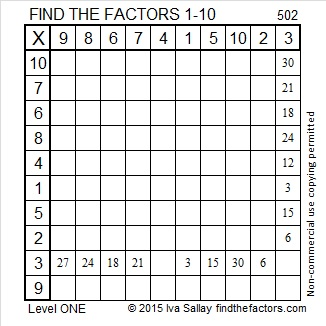# 502 and Level 1

The prime factorization of 502 is 2 x 251. How many integers less than or equal to 502 do not have either of those numbers in their prime factorizations?

There is actually a function that counts how many. It is called the totient function or Euler’s totient function and looks like φ(502).

502 is the first integer that has both 2 and 251 in its prime factorization so finding φ(502) will be easy: first eliminate the 251 integers less than or equal to 502 that are divisible by 2. Then eliminate 251 because it is the only remaining number that is divisible by 502’s other prime factor. Thus φ(502) = 502 – 251 – 1 = 250.

Notice that 502 and 250 use the same digits. I learned this fact about the number 502 and φ(502) by reading OEIS.org.Print the puzzles or type the solution on this excel file: 10 Factors 2015-05-25

—————————————————————————————————

• 502 is a composite number.
• Prime factorization: 502 = 2 x 251
• The exponents in the prime factorization are 1 and 1. Adding one to each and multiplying we get (1 + 1)(1 + 1) = 2 x 2 = 4. Therefore 502 has exactly 4 factors.
• Factors of 502: 1, 2, 251, 502
• Factor pairs: 502 = 1 x 502 or 2 x 251
• 502 has no square factors that allow its square root to be simplified. √502 ≈ 22.4053565—————————————————————————————————## 2 thoughts on “502 and Level 1”

1.Joseph Nebus

I have very few reasons to mention the “totient” function, but somehow I feel worldly when I do bring it up.

•ivasallay

I’m pretty sure this post is the very first time I have ever brought it up.

This site uses Akismet to reduce spam. Learn how your comment data is processed.